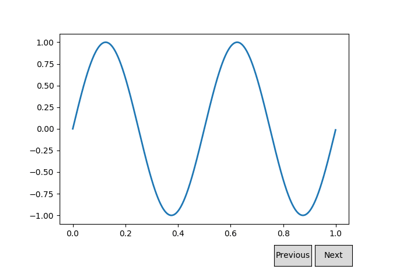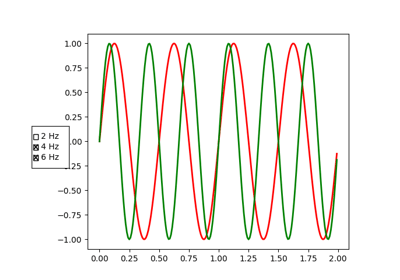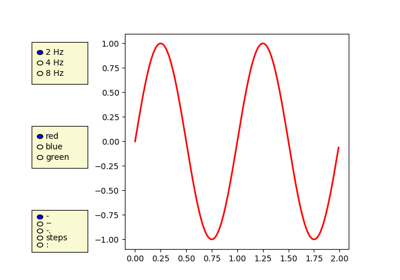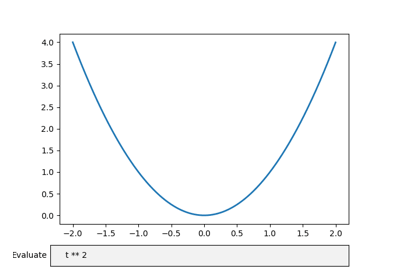/Matplotlib 3.0

# matplotlib.pyplot.draw

`matplotlib.pyplot.draw()` [source]

Redraw the current figure.

This is used to update a figure that has been altered, but not automatically re-drawn. If interactive mode is on (`ion()`), this should be only rarely needed, but there may be ways to modify the state of a figure without marking it as `stale`. Please report these cases as bugs.

A more object-oriented alternative, given any `Figure` instance, `fig`, that was created using a `pyplot` function, is:

```fig.canvas.draw_idle()
```

## Examples using `matplotlib.pyplot.draw`Interactive functionsCursor DemoRotating a 3D plotButtonsCheck Buttons